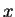# Heisenberg Effects in Computer-Arithmetic

Walter Gander, ETH Zurich

As a homework I asked my students to compute the smallest normalized floating point number. One of the student presented me this MATLAB function:

```function x = myrealmin()
x = 1;
temp = x;
while eps * temp / 2 > 0
temp = (eps * temp / 2);
if (temp > 0)
x = temp;
end
end
```
The call x = myrealmin yields the result x = 0. Why shouldbecome zero? After all the assignment x = temp is only executed when the condition temp > 0 is met! So the student wanted to debug the program by printing intermediate results. He removed the semicolon on line 5, thus stored the function
```function x = myrealmin()
x = 1;
temp = x;
while eps * temp / 2 > 0
temp = (eps * temp / 2)
if (temp > 0)
x = temp;
end
end
```
Now with the statement x = myrealmin one gets intermediate results for temp but also the final value forwhich, amazingly, is no longer zero but x = 8.0948e-320. What is going on here? A print statement for the variable temp changes the flow of a program? Are we experiencing some sort of Heisenberg effect?1Are our computer no longer deterministic machines? And do they really compute wrong? Honestly in the first case,cannot be zero, right?

A second student suggested the following MATLAB script:

```  a = 1;
while a*eps>0
last = a; a = a/2.0;
end;
a = last
```
Typed interactively in a MATLAB window this script yields the result a = 2.2251e-308. Which is really what we want since the smallest normalized real number is indeed realmin = 2.2251e-308 according to the IEEE standard for floating point numbers. However, if we write the script on a file, say on test.m and then execute the MATLAB command test then we obtain a different result a = 4.9407e-324 which is not the smallest normalized real number but the smallest denormalized number!?

What is the reason for this weird behavior? It is not a MATLAB bug. It must be explained with the fact that modern processors use 80-bit registers for processing real numbers and store results as 64-bit numbers according to the IEEE standard for double precision floating point numbers.

Beginning with MATLAB 6.5 (and continuing with MATLAB 7), test.m is compiled, that is efficient Pentium code is generated and executed. This code keeps some variables in the 80-bit registers on the chip. These have a longer fraction and a bigger exponent.

The ``Heisenberg-effect'' occurs because without intermediate printing the computation is done completely in the 80-bit registers and the final result becomes 0 due to underflow when it is stored as 64-bit floating point number.

Removing the semicolon in the second case, thus allowing printing the intermediate results, clears the registers or at least the variable temp is stored in memory so that it becomes a 64-bit floating point number.

What to do? Accept such effects and live with an unbalanced finite arithmetic? Should a new standard be defined? The discussion is open.